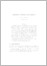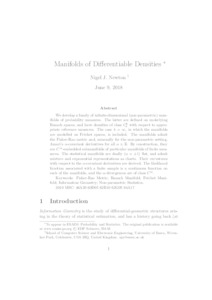# Manifolds of Differentiable Densities

Newton, NJ (2018) 'Manifolds of Differentiable Densities.' ESAIM: Probability and Statistics, 22. 19 - 34. ISSN 1262-3318Preview
Text
mosdgen_ar3.pdf - Accepted Version

We develop a family of infinite-dimensional (non-parametric) manifolds of probability measures. The latter are defined on underlying Banach spaces, and have densities of class $C_b^k$ with respect to appropriate reference measures. The case $k=\infty$, in which the manifolds are modelled on Fréchet spaces, is included. The manifolds admit the Fisher-Rao metric and, unusually for the non-parametric setting, Amari's $\alpha$-covariant derivatives for all $\alpha\in\R$. By construction, they are $C^\infty$-embedded submanifolds of particular manifolds of finite measures. The statistical manifolds are dually ($\alpha=\pm 1$) flat, and admit mixture and exponential representations as charts. Their curvatures with respect to the $\alpha$-covariant derivatives are derived. The likelihood function associated with a finite sample is a continuous function on each of the manifolds, and the $\alpha$-divergences are of class $C^\infty$.View Item